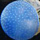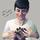## General Question# How to display all permutations of Strings in Java?

Asked by anonxd (114) April 28th, 2017

I have a question, which I want to solve myself. The question is that how to find all permutations of a String in Java. Its code is available all over internet. But I just can not understand it(to the point that I can code it myself). My brain is telling me to solve myself but this is not happening. What should I do? This is not even my assignment. This happens with a lot of problems. I don’t want the code,I already have it. But I want to know, how to tackle this kind of problem.

Observing members: 0Composing members: 0Well, how would you go about finding these permutations yourself, if you didn’t have the help of a computer program? Let’s think about this with the example of an easy, short word: test.

You might start by fixing the beginning of the word in place, and only swapping the remaining letters…

t <- fixed, “est” <- how can we swap these letters around to make different words?

Notice something here? We now need to find all the permutations of “est” – basically, we need to run the algorithm we were already trying to write, but on est instead of test. We’ve simplified the problem a little by making the word shorter. This is where we’re going to get into something called “recursion,” which is where a function calls itself on a different input until finally the input is so simple that it can figure out what to do with it. But let’s keep talking in pseudocode for now.

OK, so finding all the combinations of “est” is still pretty tough, because there’s a bunch of them, 6 I think. So let’s also fix the e in place and find all the combinations of the remaining word.

t <- fixed, e <- fixed, “st”.

Hey, finding all the combinations of st is pretty easy. There’s only st and ts. This is what we’ll call our base case, which is simple, so we don’t need to call the function again. This is how we avoid getting into an infinite loop during recursion.

t e st
t e ts

So those are all the combinations where t and e are fixed. Now we need to go back up a level to where “t” is the only fixed character, and find the other combinations of “est” that we missed.

We found all the ones where e is the first letter, so let’s make s the first letter now.

t <- fixed, s <- fixed, “et”

Again, “et” is only two letters long, so it triggers our “base case” where we know the only combinations are “et” and “te”.

t s et
t s te

So, turning this into code: if your function signature is String [] string_permutations(the_string), you’ll want to write special case for handling the case where “the_string” is only 2 letters long, which returns an array containing the_string and the_string reversed. If your string isn’t 2 letters long, fix the first letter in place and call string_permutations on the rest of the word. Eventually you’ll reach the case where the rest of the word is only 2 letters long, and then the base case will be triggered. Then, you can swap letters as needed to get the words you missed when you fixed the first characters in place. Finally, eliminate duplicates: our word “test” has two t’s, so there will be some duplicates.

Learning how to think with recursion can be tough, but it comes more easily with experience. Keep practicing, you’ll get it.

Mariah (25836)“Great Answer” (4) Flag as…There is a devilishly clever algorithm for doing permutations called the Steinhaus–Johnson–Trotter method. The basic idea is fairly simple, but it takes a little bit of work to implement. The algorithm is intended for finding permutations of numbers 1 to n. If you want to use it for a string, just treat the numbers as indices into the string.

The algorithm only swaps adjacent elements. It is best to think of it as running recursively, but the code can be implemented fairly easily without it.

Suppose we want to find permutations of the numbers 1 to 4. Start with the initial position as the first permutation of (1,2,3,4). We are going to move 4 through each permutation of (1,2,3). Moving right to left we get (1,2,4,3 ), (1,4,2,3) and (4,1,2,3). It is stuck and must change direction, but only after we get the next permutation of the numbers 1 to 3.

Now we get the next permutation of (1,2,3). We are going to move 3 through (1,2). This gives (1,3,2).

Now we can move 4 left to right through (1,3,2), giving (4,1,3,2), (1,4,3,2) and (1,3,4,2) and (1,3,2,4). 4 can’t move any further.

We switch back to 3 and again move it once. Eventually, 3 will reach the end or bump against 4. No number can move past a larger number. With 3 being stuck, we go down to 2.

To summarize, the basic idea is this:
1. Starting with n, find first number that is not stuck and transpose it with the next number.
2. Reverse direction of all the numbers that were found to be stuck.
3. Go back to step 1

At some point, all the numbers 2 to n will be stuck, at which point we are done.

Suppose that you want to find all the unique letter permutations of a word. For, example, for test, you want to treat the two t’s as equivalent. The above algorithm makes this easy. For example consider the word test. Our initial permutation is (1,2,3,4) and the indices of the two t’s are (1,4). We say that their degree of disorder is 0. Since the algorithm only transposes adjacent elements, the only way for the t’s to get out of order is if the indices 1 and 4 are being swapped. If the 4 index is going to move ahead of the 1 index, we bump the disorder by 1. Otherwise we decrement by 1. We return string permutations only when the disorder is 0. Only one disorder counter is needed for any number of instances of repeated letters.

LostInParadise (26123)“Great Answer” (0) Flag as…Response moderated (Spam)
Response moderated (Spam)

or